# RAMP on El Nino prediction

Balázs Kégl (CNRS), Claire Monteleoni (GWU), Mahesh Mohan (GWU), Timothy DelSole (COLA), Kathleen Pegion (COLA), Julie Leloup (UPMC), Alex Gramfort (LTCI), Mehdi Cherti (CNRS)

## Introduction¶

A climate index is real-valued time-series which has been designated of interest in the climate literature. For example, the El Niño–Southern Oscillation (ENSO) index has widespread uses for predictions of regional and seasonal conditions, as it tends to have strong (positive or negative) correlation with a variety of weather conditions and extreme events throughout the globe. The ENSO index is just one of the many climate indices studied. However there is currently significant room for improvement in predicting even this extremely well studied index with such high global impact. For example, most statistical and climatological models erred significantly in their predictions of the 2015 El Niño event; their predictions were off by several months. Better tools to predict such indices are critical for seasonal and regional climate prediction, and would thus address grand challenges in the study of climate change (World Climate Research Programme: Grand Challenges, 2013).

### El Niño¶

El Niño (La Niña) is a phenomenon in the equatorial Pacific Ocean characterized by a five consecutive 3-month running mean of sea surface temperature (SST) anomalies in the Niño 3.4 region that is above (below) the threshold of $+0.5^\circ$C ($-0.5\circ$C). This standard of measure is known as the Oceanic Niño Index (ONI).Mor information can be found here on why it is an important region, and what is the history of the index.

Here are the current ENSO predictions, updated monthly.

### The CCSM4 simulator¶

Our data is coming from the CCSM4.0 model (simulator). This allows us to access a full regular temperature map for a 500+ year period which makes the evaluation of the predictor more robust than if we used real measurements.

### The data¶

The data is a time series of "images" $z_t$, consisting of temperature measurements (for a technical reason it is not SST that we will work with, rather air temperature) on a regular grid on the Earth, indexed by lon(gitude) and lat(itude) coordinates. The average temperatures are recorded every month for 501 years, giving 6012 time points. The goal is to predict the temperature in the El Nino region, 6 months ahead.

Note that the data set given in this notebook may be different from the one used to evaluate your submissions (of course, the data structures will be identical), so your submission should be generic (for example, it should be able to handle a time series of different length).

Similarly to the variable stars RAMP, the pipeline will consists of a feature extractor and a predictor. Since the task is regression, the predictor will be a regressor, and the score (to minimize) will be the root mean square error. The feature extractor will have access to the whole data. It will construct a "classical" feature matrix where each row corresponds to a time point. You should collect all information into these features that you find relevant to the regressor. The feature extractor can take anything from the past, that is, it will implement a function $x_t = f(z_1, \ldots, z_t)$. Since you will have access to the full data, in theory you can cheat (even inadvertantly) by using information from the future. We have implemented a randomized test to detect such submissions, but please do your best to avoid this since it would make the results irrelevant.

### Domain-knowledge suggestions¶

You are of course free to explore any regression technique to improve the prediction. Since the input dimension is relatively large (2000+ dimensions per time point even after subsampling) sparse regression techniques (eg. LASSO) may be the best way to go, but this is just an a priori suggestion. The following list provides you other hints to start with, based on domain knowledge.

• There is a strong seasonal cycle that must be taken into account.

• There is little scientific/observational evidence that regions outside the Pacific play a role in NINO3.4 variability, so it is probably best to focus on Pacific SST for predictions.

• The relation between tropical and extra-tropical Pacific SST is very unclear, so please explore!

• The NINO3.4 index has an oscillatory character (cold followed by warm followed by cold), but this pattern does not repeat exactly. It would be useful to be able to predict periods when the oscillation is “strong” and when it “breaks down.”

• A common shortcoming of empirical predictions is that they under-predict the amplitude of warm and cold events. Can this be improved?

• There is evidence that the predictability is low when forecasts start in, or cross over, March and April (the so-called “spring barrier”). Improving predictions through the spring barrier would be important.

# Exploratory data analysis¶

Packages to install:

conda install basemap
conda install -c https://conda.binstar.org/anaconda xray
conda install netcdf4 h5py
pip install pyresample
apt-get install netcfd-bin or brew install netcdf

 
 
 
 In : %matplotlib inline from mpl_toolkits.basemap import Basemap import matplotlib.pyplot as plt import numpy as np import pandas as pd import xray # should be installed with pip import pyresample # should be installed with pip from sklearn.cross_validation import cross_val_score Let's start by reading the data into an xray Dataset object. You can find all information on how to access and manipulate Dataset and DataArray objects at the xray site. In : temperatures_xray = xray.open_dataset( 'COLA_data/tas_Amon_CCSM4_piControl_r3i1p1_000101-012012.nc', decode_times=False) # there is no way to convert a date starting with the year 800 into pd array so we # shift the starting date to 1700 temperatures_xray['time'] = pd.date_range('1/1/1700', periods=temperatures_xray['time'].shape, freq='M') - np.timedelta64(15, 'D') Printing it, you can see that it contains all the data, indices, and other metadata. In : temperatures_xray Out: <xray.Dataset> Dimensions: (bnds: 2, lat: 192, lon: 288, time: 1440) Coordinates: * time (time) datetime64[ns] 1700-01-16 1700-02-13 1700-03-16 ... * lat (lat) float64 -90.0 -89.06 -88.12 -87.17 -86.23 -85.29 -84.35 ... * lon (lon) float64 0.0 1.25 2.5 3.75 5.0 6.25 7.5 8.75 10.0 11.25 ... height float64 2.0 * bnds (bnds) int64 0 1 Data variables: time_bnds (time, bnds) float64 0.0 31.0 31.0 59.0 59.0 90.0 90.0 120.0 ... lat_bnds (lat, bnds) float64 -90.0 -89.53 -89.53 -88.59 -88.59 -87.64 ... lon_bnds (lon, bnds) float64 -0.625 0.625 0.625 1.875 1.875 3.125 ... tas (time, lat, lon) float64 246.9 247.0 246.0 246.3 247.0 246.8 ... Attributes: institution: NCAR (National Center for Atmospheric Research) Boulder, CO, USA institute_id: NCAR experiment_id: piControl source: CCSM4 model_id: CCSM4 forcing: Sl GHG SS Ds SD BC MD OC Oz AA (all fixed at 1850 values) parent_experiment_id: N/A parent_experiment_rip: N/A branch_time: 0.0 contact: cesm_data@ucar.edu comment: CESM home page: http://www.cesm.ucar.edu references: Gent P. R., et.al. 2011: The Community Climate System Model version 4. J. Climate, doi: 10.1175/2011JCLI4083.1 initialization_method: 1 physics_version: 1 tracking_id: 818f2df4-f49b-480a-91aa-a6eb9c5534c2 acknowledgements: The CESM project is supported by the National Science Foundation and the Office of Science (BER) of the U.S. Department of Energy. NCAR is sponsored by the National Science Foundation. This research used resources of the National Energy Research Scientific Computing Center, which is supported by the Office of Science (BER) of the U.S. Department of Energy under Contract No. DE-AC02-05CH11231 cesm_casename: b40.pictrl_cosp.cam4.1deg.001 cesm_repotag: cesm1_0_beta20 cesm_compset: B1850CN resolution: f09_g16 (0.9x1.25_gx1v6) forcing_note: Additional information on the external forcings used in this experiment can be found at http://www.cesm.ucar.edu/CMIP5/forcing_information processed_by: strandwg on mirage4 at 20120706 -192826.864 processing_code_information: Last Changed Rev: 1030 Last Changed Date: 2012-07-06 09:35:11 -0600 (Fri, 06 Jul 2012) Repository UUID: d2181dbe-5796-6825-dc7f-cbd98591f93d product: output experiment: pre-industrial control frequency: mon creation_date: 2012-07-07T01:28:27Z history: 2012-07-07T01:28:27Z CMOR rewrote data to comply with CF standards and CMIP5 requirements. Conventions: CF-1.4 project_id: CMIP5 table_id: Table Amon (12 January 2012) 4996d487f7a65749098d9cc0dccb4f8d title: CCSM4 model output prepared for CMIP5 pre-industrial control parent_experiment: N/A modeling_realm: atmos realization: 3 cmor_version: 2.8.1 The main data is in the 'tas' ("temperature at surface") DataArray. In : temperatures_xray['tas'] Out: <xray.DataArray 'tas' (time: 1440, lat: 192, lon: 288)> [79626240 values with dtype=float64] Coordinates: * time (time) datetime64[ns] 1700-01-16 1700-02-13 1700-03-16 ... * lat (lat) float64 -90.0 -89.06 -88.12 -87.17 -86.23 -85.29 -84.35 ... * lon (lon) float64 0.0 1.25 2.5 3.75 5.0 6.25 7.5 8.75 10.0 11.25 ... height float64 2.0 Attributes: standard_name: air_temperature long_name: Near-Surface Air Temperature units: K original_name: TREFHT comment: TREFHT no change cell_methods: time: mean (interval: 30 days) cell_measures: area: areacella history: 2012-07-07T01:28:26Z altered by CMOR: Treated scalar dimension: 'height'. 2012-07-07T01:28:26Z altered by CMOR: Reordered dimensions, original order: lat lon time. associated_files: baseURL: http://cmip-pcmdi.llnl.gov/CMIP5/dataLocation gridspecFile: gridspec_atmos_fx_CCSM4_piControl_r0i0p0.nc areacella: areacella_fx_CCSM4_piControl_r0i0p0.nc 
 
 
 

You can index it in the same way as a pandas or numpy array. The result is always a DataArray

In :
t = 123
lat = 13
lon = 29
temperatures_xray['tas'][t]
temperatures_xray['tas'][t, lat]
temperatures_xray['tas'][t, lat, lon]
temperatures_xray['tas'][:, lat, lon]
temperatures_xray['tas'][t, :, lon]
temperatures_xray['tas'][:, :, lon]

Out:
<xray.DataArray 'tas' (time: 1440, lat: 192)>
[276480 values with dtype=float64]
Coordinates:
* time     (time) datetime64[ns] 1700-01-16 1700-02-13 1700-03-16 ...
* lat      (lat) float64 -90.0 -89.06 -88.12 -87.17 -86.23 -85.29 -84.35 ...
lon      float64 36.25
height   float64 2.0
Attributes:
standard_name: air_temperature
long_name: Near-Surface Air Temperature
units: K
original_name: TREFHT
comment: TREFHT no change
cell_methods: time: mean (interval: 30 days)
cell_measures: area: areacella
history: 2012-07-07T01:28:26Z altered by CMOR: Treated scalar dimension: 'height'. 2012-07-07T01:28:26Z altered by CMOR: Reordered dimensions, original order: lat lon time.
associated_files: baseURL: http://cmip-pcmdi.llnl.gov/CMIP5/dataLocation gridspecFile: gridspec_atmos_fx_CCSM4_piControl_r0i0p0.nc areacella: areacella_fx_CCSM4_piControl_r0i0p0.nc

You can convert any of these objects into a numpy array.

In :
temperatures_xray['tas'].values
temperatures_xray['tas'][t].values
temperatures_xray['tas'][t, lat].values
temperatures_xray['tas'][t, lat, lon].values

Out:
array(218.4674835205078)

You can also use slices, and slice bounds don't even have to be in the index arrays. The following function computes the target at time $t$. The input is an xray DataArray (3D panel) that contains the temperatures. We select the El Nino 3.4 region, and take the mean temperatures, specifying that we are taking the mean over the spatial (lat and lon) coordinates. The output is a vector with the same length as the original time series.

In :
en_lat_bottom = -5
en_lat_top = 5
en_lon_left = 360 - 170
en_lon_right = 360 - 120

def get_area_mean(tas, lat_bottom, lat_top, lon_left, lon_right):
"""The array of mean temperatures in a region at all time points."""
return tas.loc[:, lat_bottom:lat_top, lon_left:lon_right].mean(dim=('lat','lon'))

def get_enso_mean(tas):
"""The array of mean temperatures in the El Nino 3.4 region at all time points."""
return get_area_mean(tas, en_lat_bottom, en_lat_top, en_lon_left, en_lon_right)


The following function plots the temperatures at a given $t$ (time_index).

In :
el_nino_lats = [en_lat_bottom, en_lat_top, en_lat_top, en_lat_bottom]
el_nino_lons = [en_lon_right, en_lon_right, en_lon_left, en_lon_left]

from matplotlib.patches import Polygon

def plot_map(temperatures_xray, time_index):
def draw_screen_poly(lats, lons, m):
x, y = m(lons, lats)
xy = zip(x,y)
poly = Polygon(xy, edgecolor='black', fill=False)

lons, lats = np.meshgrid(temperatures_xray['lon'], temperatures_xray['lat'])

fig = plt.figure()
map = Basemap(llcrnrlon=0, llcrnrlat=-89, urcrnrlon=360, urcrnrlat=89, projection='mill')
# draw coastlines, country boundaries, fill continents.
map.drawcoastlines(linewidth=0.25)
#map.drawcountries(linewidth=0.25)
#map.fillcontinents(color='coral',lake_color='aqua')
# draw the edge of the map projection region (the projection limb)
#map.drawmapboundary(fill_color='aqua')
im = map.pcolormesh(lons, lats, temperatures_xray[time_index] - 273.15,
draw_screen_poly(el_nino_lats, el_nino_lons, map)

time_str = str(pd.to_datetime(str(temperatures_xray['time'].values[time_index])))[:7]
ax.set_title("Temperature map " + time_str)
#plt.savefig("test_plot.pdf")
plt.show()


Let's plot the temperature at a given time point. Feel free to change the time, play with the season, discover visually the variability of the temperature map.

In :
t = 12
plot_map(temperatures_xray['tas'], t)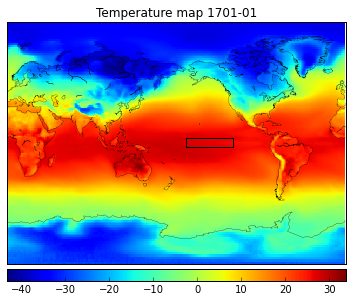## The target¶

The first order variation of the temperature comes from its regular yearly fluctuation. Climate scientist usually look at the temperture anomaly, not the raw temperature itself. More precisely, they subtract the montly average from the temperature. We set up the RAMP to predict the raw temperature because 1) subtracting the montly average is equivalent to adding the monthly average as an input feature to the regressor 2) we wanted to avoid computing the average on the full data since it would have violated causality (remember: you are not allowed to use the future, not even for computing averages). Nevertheless, it is interesting to look at the anomaly since you can compare it to plots produced by climate scientist.

The snippet also shows some powerful features of xray (grouping by months, taking the groupby mean).

In :
enso = get_enso_mean(temperatures_xray['tas'])
enso_anomaly = enso.groupby('time.month') - enso.groupby('time.month').mean(dim='time')


We plot the anomaly in a five year period. Conventionally, the El Nino/La Nina threshold is $0.5^\circ$ Celsius. The plot displays the warm periods as red (El Nino) and cold perids (La Nina) as blue. To be precise, El Nino requires a period of consecutive three months with mean temperatures $0.5^\circ$ Celsius above the seasonal average, so not all colored periods qualify.

In :
plt.clf()
xrange = np.arange(0,600)
y = enso_anomaly[600:1200]
x_limits = [min(xrange), max(xrange)]
y_limits = [-3.0, 3.0]
el_nino_threshold = 0.5
plt.xlim(x_limits)
plt.ylim(y_limits)
plt.plot(xrange, y,c='black')
plt.plot(x_limits, [el_nino_threshold, el_nino_threshold], c='r')
plt.plot(x_limits, [-el_nino_threshold, -el_nino_threshold], c='b')
plt.fill_between(xrange, el_nino_threshold, y, color='red', where=y>=el_nino_threshold, interpolate=True)
plt.fill_between(xrange, -el_nino_threshold, y, color='blue', where=y<=-el_nino_threshold)
plt.savefig("anomaly.png")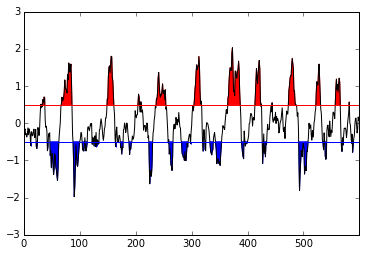## Downsampling¶

In principle we could keep the full temperature map with its 55296 values, and let you take care of the sparsification and subsampling. We found that this would unnecessarily overwhelm the system (especially the memory), so we decided to downsample the temperature grid from a $1^\circ$ resolution to a $5^\circ$ resolution, using the pyresample package.

In :
lat_bins = np.arange(-90,91,5, dtype=float)
lon_bins = np.arange(2.5,360,5, dtype=float)

In :
lat_in, lon_in = np.meshgrid(temperatures_xray['lat'], temperatures_xray['lon'])
grid_in = pyresample.geometry.SwathDefinition(lats=lat_in, lons=lon_in - 180)
lat_out, lon_out = np.meshgrid(lat_bins, lon_bins)
grid_out = pyresample.geometry.SwathDefinition(lats=lat_out, lons=lon_out - 180)


The following cell will take some time. You can altternatively load the downsampled file below.

resampled_tas = np.empty((temperatures_xray['tas'].shape, lat_bins.shape, lon_bins.shape), dtype=float) for t in range(temperatures_xray['tas'].shape): print t resampled_tas[t] = pyresample.kd_tree.resample_nearest( grid_in, temperatures_xray['tas'][t].values.T, grid_out, radius_of_influence=500000, fill_value=None).data.Tresampled_tas.shaperesampled_xray = xray.Dataset( {'tas': (['time', 'lat', 'lon'], resampled_tas)}, coords={'time': temperatures_xray['time'], 'lat': lat_bins, 'lon': lon_bins})

Alternatively, if you have saved the file below, you can reload it here.

In :
resampled_xray = xray.open_dataset(
'COLA_data/resampled_tas_Amon_CCSM4_piControl_r3i1p1_000101-012012.nc', decode_times=False)

In :
t = -12
plot_map(resampled_xray['tas'], t)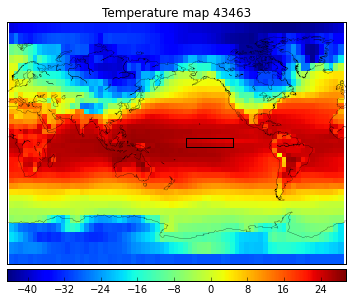## The cross-validation object¶

Cross validating time-series predictors is tricky. We can't simply shuffle the observations $z_t =$ temperatures_xray[t] since we would lose both causality and the correlation structure that follows natural order.

To formalize the issue, let us first define formally the predictor that we will produce in the RAMP. Let the time series (of images) be $z_1, \ldots, z_T$ and the let target to predict at time $t$ be $y_t$. The target is usually (and in our case) a function of the future $z_{t+1}, \ldots$, but it can be anything else. We want to learn a function that predicts $y$ from the past, that is

\begin{equation} \hat{y}_t = f(z_1, ..., z_t) = f(Z_t) \end{equation}

where $Z_t = (z_1, ..., z_t)$ is the past. Now, the sample $(Z_t, y_t)$ is a regular (although none iid) sample from the point of view of shuffling, so we can train on $\{Z_t, y_t\}_{t \in \cal{I}_{\text{train}}}$ and test on $(Z_t, y_t)_{t \in \cal{I}_{\text{test}}}$, where $\cal{I}_{\text{train}}$ and $\cal{I}_{\text{test}}$ are arbitrary but disjunct train and test index sets, respectively (typically produced by sklearn's ShuffleSplit).

The training algorithm thus maps $(Z_t, y_t)_{t \in \cal{I}_{\text{train}}}$ to $f$. The training $Z_t$s overlap, so basically the module will have to have access to all the data $Z_T$ and training labels $\{y_t\}_{t \in \cal{I}_{\text{train}}}$. You will have to be careful produce a predictor $f$ that of course can only use the past at test time.

To allow a reasonably long past before making the first prediction, we strip the first $b = 120$ months (burn-in). You can of course use a longer window in your feature extractor, but in this case you will have to handle the missing time points in the beginning of the sequence. We also cannot use the last six months since we don't have a target for these.

In :
from sklearn.cross_validation import ShuffleSplit

random_state = 61
n_burn_in = 120
skf = ShuffleSplit(resampled_xray['time'].shape - n_burn_in - n_lookahead, n_iter=2,
test_size=0.5, random_state=random_state)


## The target¶

The goal is to predict the temperature in the El Nino region, $\ell = 6$ months ahead. For this we have to shift the enso vector by six months to the right, or equivalently, its time index by six months to the left. We then add the target as a new DataArray into the temperatures_xray Dataset, so that the feature extractor can access it. The formal definition of the target is

\begin{equation} y_t = \frac{1}{D} \sum_{(lat, lon) \in \cal{A}_{\rm{El Nino}}} z_{t + \ell}^{(lat, lon)} \end{equation}

Where $\cal{A}_{\rm{El Nino}} = \{(lat, lon) : -5^\circ < lat < 5^\circ \wedge -170^\circ < lon < -120^\circ\}$ is the index set of the El Nino 3.4 region, $D$ is the number of temperature measurements in the region, and $z_t^{(lat, lon)}$ is the temperature measured at time $t$, longitude $lon$ and latitude $lat$.

If you reloaded resampled_xray above, don't execute this (resampled_xray contains the target).

target = enso.copy(deep=True) target['time'] = np.roll(target['time'], n_lookahead) target[:n_lookahead] = np.NaN resampled_xray['target'] = target

You can save resampled_xray here.

resampled_xray.to_netcdf('COLA_data/resampled_tas_Amon_CCSM4_piControl_r3i1p1_000101-012012.nc')

valid_range is the index set that indicates the time points where a prediction should be made. y is the set of targets in this range.

In :
valid_range = range(n_burn_in, resampled_xray['time'].shape - n_lookahead)
y = resampled_xray['target'][valid_range].values
y

Out:
array([ 299.17263526,  298.18955123,  298.03081985, ...,  298.9415176 ,
299.29809086,  299.51065049])

The following shows that the target varies around its mean at about $0.97^\circ$C, which is a thus a rough upper bound on the score of the simplest predictor.

In :
print y.mean()
print y.std()
plt.hist(y)

298.914621414
0.97287968808

Out:
(array([   4.,    5.,   15.,   33.,  141.,  281.,  353.,  285.,  163.,   34.]),
array([ 294.62714472,  295.2910842 ,  295.95502368,  296.61896317,
297.28290265,  297.94684214,  298.61078162,  299.27472111,
299.93866059,  300.60260008,  301.26653956]),
<a list of 10 Patch objects>)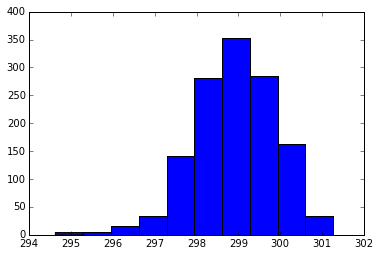To make a tighter upper bound, let's look at the standard deviation of the seasonally adjusted temperature.

In :
print enso_anomaly.values.mean()
print enso_anomaly.values.std()
plt.hist(enso_anomaly);

7.57912251477e-15
0.863325139094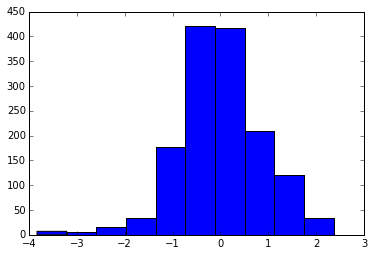## The pipeline¶

We have factorized the pipeline into two steps. The first feature extractor $g$ transforms the past into a classical feature vector $x_t = g(Z_t)$, and the classical regressor $h$ predicts the target from the feature vector $\hat{y}_t = h(x_t)$. To summarize, the full predictor is a composition $f(Z_t) = h(g(Z_t))$. If you have a complex solution where this factorization does not make sense, you can do all the work in the feature extractor, output a prediction $x_t$ into a single-column feature matrix, and then use an identity regressor $\hat{y}_t = x_t$ in the regressor module. This setup cannot handle sophisticated techniques that do not separate feature extraction from regression (like RNNs), but it is a fundamental issue (we do not know how to bootstrap an RNN).

### The feature extractor¶

The feature extractor implements a single transform function. As we explained above, it receives the full temperaturesxray, the length of the burn-in period $b$, the look-ahead $\ell$, and the CV object skf_is = (train_is, test_is). It should produce a feature matrix of length $T - b - \ell$ representing the past vector $(Z{t+b}, \ldots, Z_{T-\ell})$. For constructing/computing $x_t$, it can only use the past $Z_t = (z_1, \ldots, z_t) =$ temperatures_xray['tas'][:t].

You can choose one of the example feature extractors and copy-paste it into your ts_feature_extractor.py file. Comments within the cells explain what they do.

In :
import numpy as np

en_lat_bottom = -5
en_lat_top = 5
en_lon_left = 360 - 170
en_lon_right = 360 - 120

def get_area_mean(tas, lat_bottom, lat_top, lon_left, lon_right):
"""The array of mean temperatures in a region at all time points."""
return tas.loc[:, lat_bottom:lat_top, lon_left:lon_right].mean(dim=('lat','lon'))

def get_enso_mean(tas):
"""The array of mean temperatures in the El Nino 3.4 region at all time points."""
return get_area_mean(tas, en_lat_bottom, en_lat_top, en_lon_left, en_lon_right)

class FeatureExtractor(object):

def __init__(self):
pass

def transform(self, temperatures_xray, n_burn_in, n_lookahead, skf_is):
"""Compute the single variable of mean temperatures in the El Nino 3.4
region."""
# This is the range for which features should be provided. Strip
# the burn-in from the beginning and the prediction look-ahead from
# the end.
valid_range = range(n_burn_in, temperatures_xray['time'].shape - n_lookahead)
enso = get_enso_mean(temperatures_xray['tas'])
enso_valid = enso.values[valid_range]
X = enso_valid.reshape((enso_valid.shape, 1))
return X

In :
import numpy as np

en_lat_bottom = -5
en_lat_top = 5
en_lon_left = 360 - 170
en_lon_right = 360 - 120

def get_enso_mean(tas):
"""The array of mean temperatures in the El Nino 3.4 region at all time points."""
return tas.loc[:, en_lat_bottom:en_lat_top, en_lon_left:en_lon_right].mean(dim=('lat','lon'))

class FeatureExtractor(object):

def __init__(self):
pass

pass

def transform(self, temperatures_xray, n_burn_in, n_lookahead, skf_is):
"""Compute the single variable of montly means corresponding to the temperatures in the El Nino 3.4
region."""
# This is the range for which features should be provided. Strip
# the burn-in from the beginning and the prediction look-ahead from
# the end.
valid_range = range(n_burn_in, temperatures_xray['time'].shape - n_lookahead)
enso = get_enso_mean(temperatures_xray['tas'])
# reshape the vector into a table years as rows, months as columns
enso_matrix = enso.values.reshape((-1,12))
count_matrix = np.ones(enso_matrix.shape)
# compute cumulative means of columns (remember that you can only use
# the past at each time point) and reshape it into a vector
enso_monthly_mean = (enso_matrix.cumsum(axis=0) / count_matrix.cumsum(axis=0)).ravel()
# roll it backwards (6 months) so it corresponds to the month of the target
enso_monthly_mean_rolled = np.roll(enso_monthly_mean, n_lookahead - 12)
# select valid range
enso_monthly_mean_valid = enso_monthly_mean_rolled[valid_range]
# reshape it into a matrix of a single column
X = enso_monthly_mean_valid.reshape((enso_monthly_mean_valid.shape, 1))
return X

In :
import numpy as np

en_lat_bottom = -5
en_lat_top = 5
en_lon_left = 360 - 170
en_lon_right = 360 - 120

def get_enso_mean(tas):
"""The array of mean temperatures in the El Nino 3.4 region at all time points."""
return tas.loc[:, en_lat_bottom:en_lat_top, en_lon_left:en_lon_right].mean(dim=('lat','lon'))

class FeatureExtractor(object):

def __init__(self):
pass

def transform(self, temperatures_xray, n_burn_in, n_lookahead, skf_is):
"""Combine two variables: the montly means corresponding to the month of the target and
the current mean temperature in the El Nino 3.4 region."""
# This is the range for which features should be provided. Strip
# the burn-in from the beginning and the prediction look-ahead from
# the end.
valid_range = range(n_burn_in, temperatures_xray['time'].shape - n_lookahead)
enso = get_enso_mean(temperatures_xray['tas'])
# reshape the vector into a table years as rows, months as columns
enso_matrix = enso.values.reshape((-1,12))
count_matrix = np.ones(enso_matrix.shape)
# compute cumulative means of columns (remember that you can only use
# the past at each time point) and reshape it into a vector
enso_monthly_mean = (enso_matrix.cumsum(axis=0) / count_matrix.cumsum(axis=0)).ravel()
# roll it backwards (6 months) so it corresponds to the month of the target
enso_monthly_mean_rolled = np.roll(enso_monthly_mean, n_lookahead - 12)
# select valid range
enso_monthly_mean_valid = enso_monthly_mean_rolled[valid_range]
enso_valid = enso.values[valid_range]
X = np.array([enso_valid, enso_monthly_mean_valid]).T
return X

In :
class FeatureExtractor(object):

def __init__(self):
pass

pass

def transform(self, temperatures_xray, n_burn_in, n_lookahead, skf_is):
"""Use world temps as features."""
# Set all temps on world map as features
all_temps = temperatures_xray['tas'].values
time_steps, lats, lons = all_temps.shape
all_temps = all_temps.reshape((time_steps,lats*lons))

return all_temps


### The regressor¶

The regressor should implement an sklearn-like regressor with fit and predict functions. Let's start with two simple regressors, a linear regressor and a regression tree. You can copy paste either of these into your first regressor.py file.

In :
from sklearn.linear_model import LinearRegression
from sklearn.base import BaseEstimator

class Regressor(BaseEstimator):
def __init__(self):
self.clf = LinearRegression()

def fit(self, X, y):
self.clf.fit(X, y)

def predict(self, X):
return self.clf.predict(X)

In :
from sklearn.tree import DecisionTreeRegressor
from sklearn.base import BaseEstimator

class Regressor(BaseEstimator):
def __init__(self):
self.clf = DecisionTreeRegressor(max_depth=5)

def fit(self, X, y):
self.clf.fit(X, y)

def predict(self, X):
return self.clf.predict(X)


### Unit test¶

This cell unit-tests your selected regressor and feature extractor and prints the RMSE. Please run it before submitting.

In :
valid_range = range(n_burn_in, resampled_xray['time'].shape - n_lookahead)
y_array = resampled_xray['target'][valid_range].values
y = y_array.reshape((y_array.shape, 1))
fe = FeatureExtractor()
X = fe.transform(resampled_xray, n_burn_in, n_lookahead, list(skf))
reg = Regressor()
scores = np.sqrt(-cross_val_score(reg, X=X, y=y, scoring='mean_squared_error', cv=skf))
print scores.mean()

0.859838484458


Since the feature extractor receives the full data series which contains the target, we have no technical constraints against cheating, that is, using information coming after the present (six months before the target). We can nevertheless check wether the feature $x_t$ changes if we alter the future $(z_{t+1}, \ldots)$. Such check is implemented below (so you can check if your submission is valid). A similar check will be run on your submissions, so you will receive a similar error message on the leaderboard if your model doesn't pass the test.

Of course, you have no reason to deliberately cheat, but it is possible that you accidentally introduce a bug. For example, the code below rolls the features in the wrong way (backward instead of forward):
enso_anomaly_rolled = np.roll(enso_anomaly, n_lookahead - 12,axis = 0)

In :
import numpy as np
from scipy import signal

en_lat_bottom = -5
en_lat_top = 5
en_lon_left = 360 - 170
en_lon_right = 360 - 120

en_f_lat_bottom = -10
en_f_lat_top = 10
en_f_lon_left = 160
en_f_lon_right = 270

def get_enso_mean(tas):
return tas.loc[:, en_lat_bottom:en_lat_top, en_lon_left:en_lon_right].mean(dim=('lat','lon'))

def select_box(tas):
return tas.loc[:, en_f_lat_bottom:en_f_lat_top, en_f_lon_left:en_f_lon_right]

def reshape_tas(tas):
#
# Get n_time , n_long , n_lat
theShape = tas.shape
n_time,n_lat,n_long = theShape,theShape,theShape
print n_time,n_lat,n_long

return tas.values.reshape(-1,12,n_lat,n_long)

#return tas.loc[:, en_f_lat_bottom:en_f_lat_top, en_f_lon_left:en_f_lon_right]

#apply reshape

#reshaped_tas = reshape_tas(selected_tas)

#plt.plot(reshaped_xray['tas'][1:36,30,37])

#plt.plot(selected_tas[1:360,30,11])
#plt.plot(reshaped_tas[1:10,:,-1,11].ravel())

#print reshaped_tas.shape

class FeatureExtractor(object):

def __init__(self):
pass

pass

def transform(self, resampled_xray, n_burn_in, n_lookahead, skf_is):
"""Use world temps as features."""
# Set all temps on world map as features
#valid_range = range(n_burn_in, temperatures_xray['time'].shape - n_lookahead)
#time_steps, lats, lons = temperatures_xray['tas'].values.shape
#X = temperatures_xray['tas'].values.reshape((time_steps,lats*lons))
#X = X[valid_range,:]

tas = select_box(resampled_xray['tas'])

valid_range = range(n_burn_in, resampled_xray['time'].shape - n_lookahead)
#enso = get_enso_mean(temperatures_xray['tas'])
# reshape the vector into a table years as rows, months as columns
#enso_matrix = enso.values.reshape((-1,12))

theShape = tas.shape
n_time,n_lat,n_long = theShape,theShape,theShape
#print n_time,n_lat,n_long
enso_matrix = tas.values.reshape(-1,12,n_lat,n_long)

count_matrix = np.ones(enso_matrix.shape)
# compute cumulative means of columns (remember that you can only use
# the past at each time point) and reshape it into a vector
enso_monthly_mean = (enso_matrix.cumsum(axis=0) / count_matrix.cumsum(axis=0)).reshape(-1,n_lat,n_long)#.ravel()
# roll it backwards (6 months) so it corresponds to the month of the target

enso_anomaly = tas - enso_monthly_mean

# rolling in the wrong direction
enso_anomaly_rolled = np.roll(enso_anomaly, n_lookahead - 12,axis = 0)
# select valid range
enso_anomaly_rolled_valid = enso_anomaly_rolled[valid_range,:,:]
# reshape it into a matrix of a single column
X = enso_anomaly_rolled_valid.reshape(-1,n_lat*n_long)

return X


The check below throws an exception stating the number of months by which the feature extractor looks into the future. If the submission passes the check, nothing happens.

In :
def check_model(X_xray, cv_is):
check_index = 200
fe = FeatureExtractor()
X_array = fe.transform(X_xray, n_burn_in, n_lookahead, cv_is)
X1 = fe.transform(X_xray, n_burn_in, n_lookahead, cv_is)
check_xray = X_xray.copy(deep=True)
check_xray['tas'][n_burn_in + check_index:] += \
np.random.normal(0.0, 10.0, check_xray['tas'][n_burn_in + check_index:].shape)
X2 = fe.transform(check_xray, n_burn_in, n_lookahead, cv_is)
first_modified_index = np.argmax(np.not_equal(X1, X2)[:,0])
if first_modified_index < check_index:
message = "The feature extractor looks into the future by {} months".format(
check_index - first_modified_index)
raise AssertionError(message)

In :
check_model(resampled_xray, list(skf))

---------------------------------------------------------------------------
AssertionError                            Traceback (most recent call last)
<ipython-input-30-415f9dbfe62f> in <module>()
----> 1 check_model(resampled_xray, list(skf))

<ipython-input-29-6e6c63b930ea> in check_model(X_xray, cv_is)
11         message = "The feature extractor looks into the future by {} months".format(
12             check_index - first_modified_index)
---> 13         raise AssertionError(message)

AssertionError: The feature extractor looks into the future by 6 months

## Submission¶

For submitting your code, first create a github repo and enter its public address (https://...) at the submission site. Place your to files regressor.py, and ts_feature_extractor.py in the git repo, and then run the following sequence.

 git add regressor.py ts_feature_extractor.py git commit -m "my commit log" git tag model_description git push origin master --tags 

We will fetch your submission. At this point it will appear in the "New models" table. Once it is trained, it will either be added to the leaderboards, or it will appear in the "Failed models" table. Clicking on “error” will bring you to the error that python threw.

### Deleting failed models¶

You cannot delete models once they appear in the leaderboard. However, you can delete failed models by executing the following sequence:

 git tag -d model_description git push origin :refs/tags/model_description 

The first command deletes your local tag and the second command deletes the remote tag. When we fetch next time, the model will disappear from the table. You can then reuse the tag to resubmit another model.